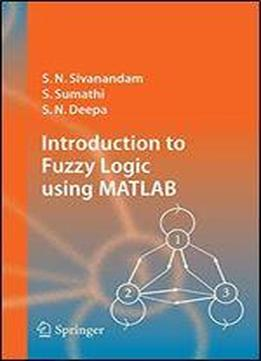# Introduction To Fuzzy Logic Using MatlabTags: S. Sumathi

This book provides a broad-ranging, but detailed overview of the basics of Fuzzy Logic. The fundamentals of Fuzzy Logic are discussed in detail, and illustrated with various solved examples. The book also deals with applications of Fuzzy Logic, to help readers more fully understand the concepts involved. Solutions to the problems are programmed using MATLAB 6.0, with simulated results. The MATLAB Fuzzy Logic toolbox is provided for easy reference.